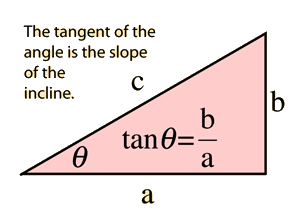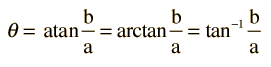Right Triangle RelationshipsThe hypotenuse of a right triangle is related to the sides by the Pythagorean relationship:From the two sides, either of the two acute angles may be found:Given any two sides, the angles may be found using the triangle trigonometric relationships. If the hypotenuse and one side are known, the other side may be calculated from:Index

 HyperPhysics*****HyperMath*****Geometry R Nave
Go Back

Equation of a LineIndex

 HyperPhysics*****HyperMath*****Geometry R Nave
Go Back